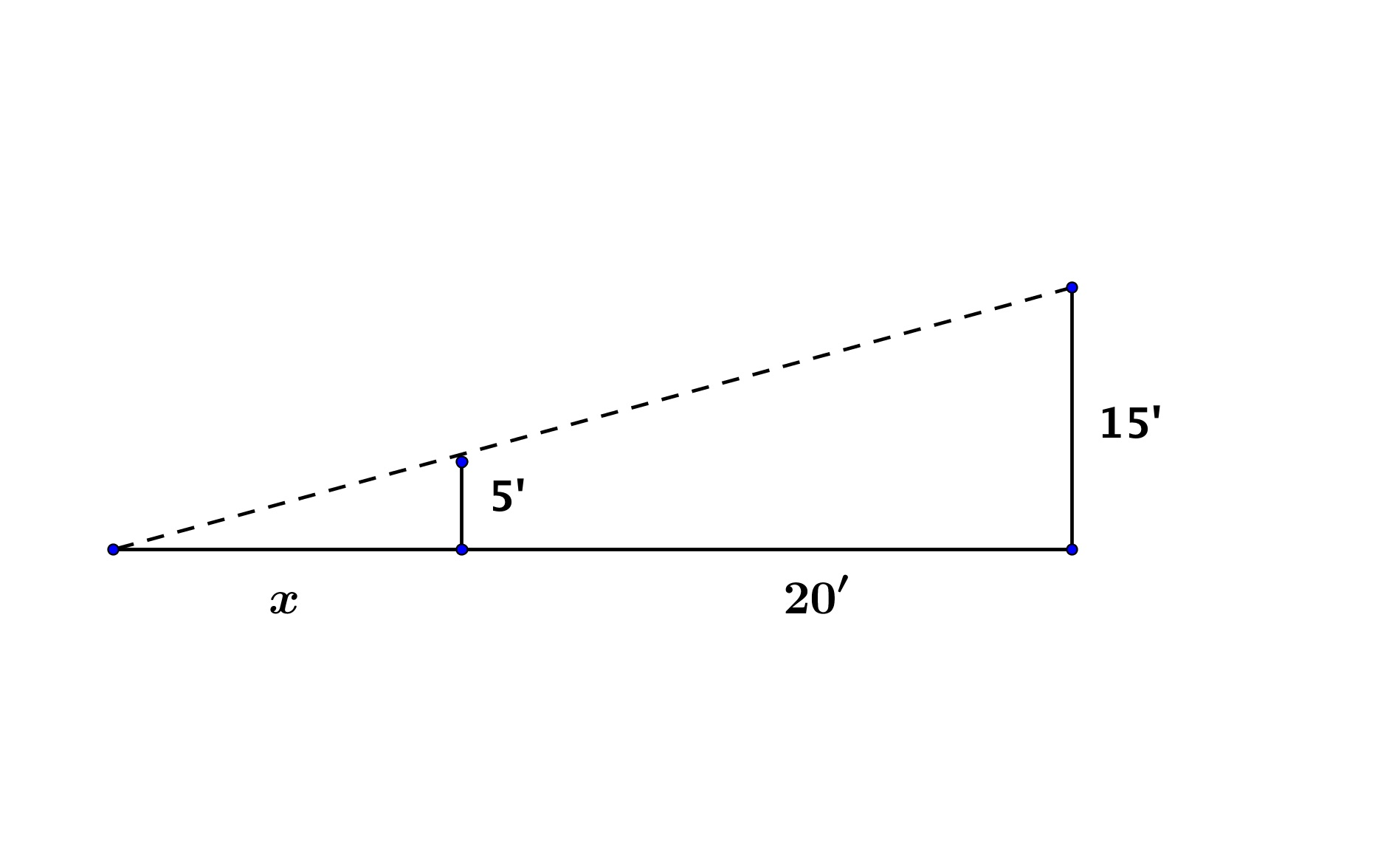# GEOM 3 | Lesson 4 | Making Connections1).  Your friend, Misha, solved the problem above by first setting up the proportion:$$\Large\frac{x}{20}= \Large\frac{5}{15}$$ and found that $$x = 6\frac{2}{3}’$$.  Your other friend, Jon, set up the proportion: $$\Large\frac{5}{15}=\Large\frac{x}{x+20}$$ and found that $$x = 10’$$.  Who is correct and why?

2).  Think of another application, create a problem around it and solve it.